# Graphing in Science XKCD Graphing is important in

• Slides: 14Graphing in Science XKCDGraphing is important in science because: 1. allows you to visualize data 2. allows for comparison of experimental results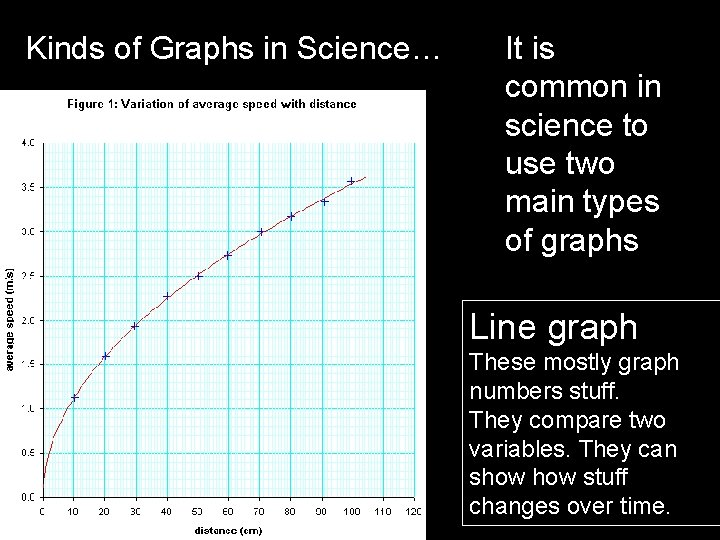Kinds of Graphs in Science… It is common in science to use two main types of graphs Line graph These mostly graph numbers stuff. They compare two variables. They can show stuff changes over time.Kinds of Graphs in Science… It is common in science to use two main types of graphs Bar graph They make comparisons between different variables very easy to see.Graphing basics… 1. Graphs have an X and Y axis The Y axis is the Vertical axis. . Just remember… a Y needs a leg to stand on Y The X axis is the horizontal axis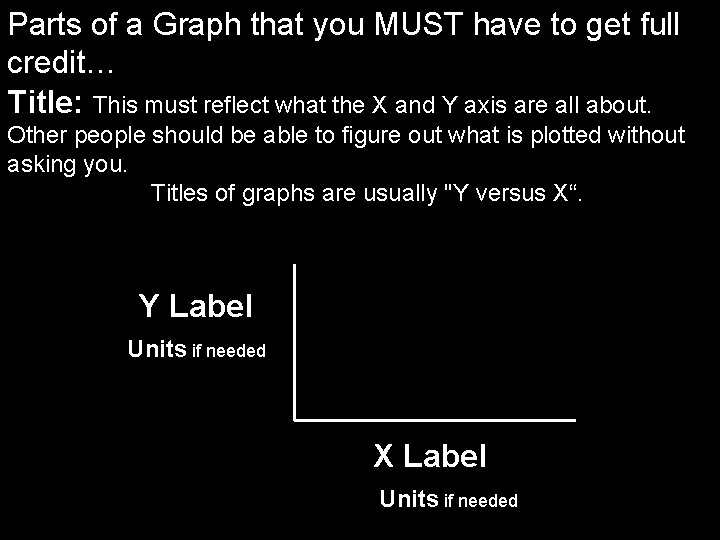Parts of a Graph that you MUST have to get full credit… Title: This must reflect what the X and Y axis are all about. Other people should be able to figure out what is plotted without asking you. Titles of graphs are usually "Y versus X“. Y Label Units if needed X Label Units if needed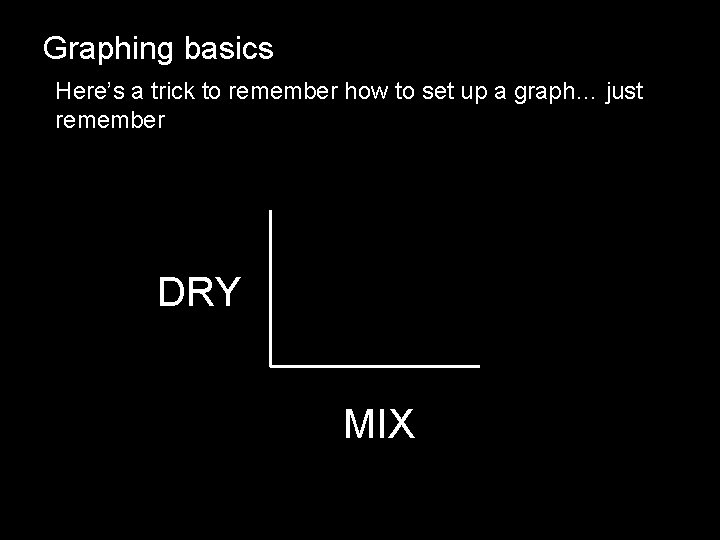Graphing basics Here’s a trick to remember how to set up a graph… just remember DRY MIX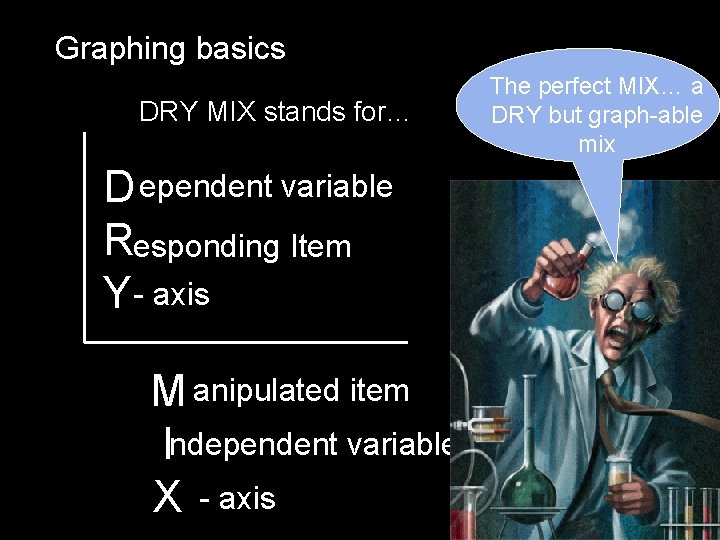Graphing basics DRY MIX stands for… D ependent variable Responding Item Y- axis M anipulated item Independent variable X - axis The perfect MIX… a DRY but graph-able mixGraphing basics Number of contaminatesvolcanic lake Lets practice determining independent and dependent variables Amount of lead in the soil- landfill locations Number of math problems Homework assignment IQ points- Shoe size Planets – solar systemGraphing basics… Let’s practice A student conducts an experiment and collects the following data… Let’s PRACTICE!!!! Homework problems assigned Time in hours 10 problems 1 25 problems 2 5 problems 3 35 problems 4 15 problems 5 How would we put this data into graph form?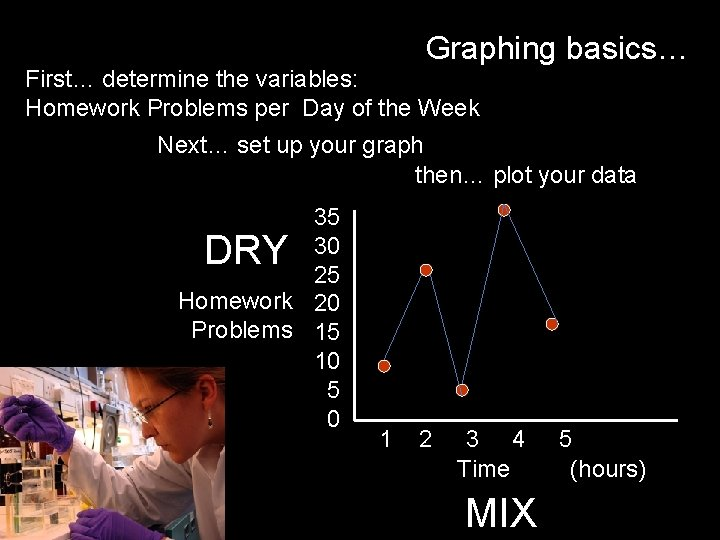Graphing basics… First… determine the variables: Homework Problems per Day of the Week Next… set up your graph then… plot your data 35 30 25 Homework 20 Problems 15 10 5 0 DRY 1 2 3 4 Time MIX 5 (hours)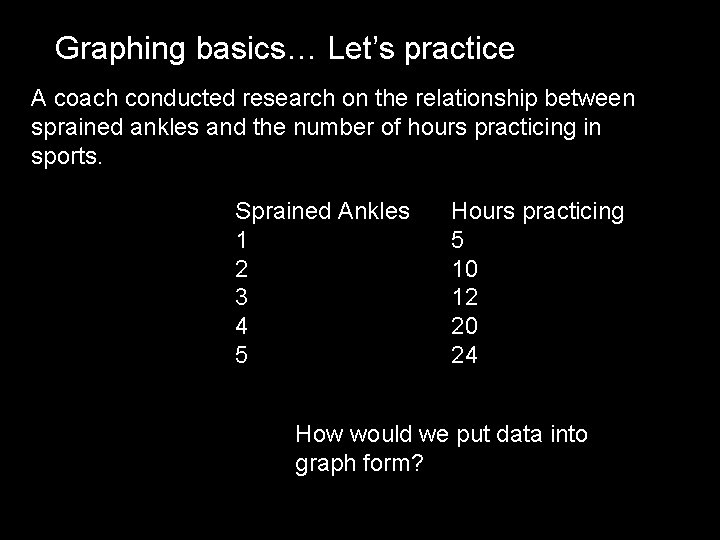Graphing basics… Let’s practice A coach conducted research on the relationship between sprained ankles and the number of hours practicing in sports. Sprained Ankles 1 2 3 4 5 Hours practicing 5 10 12 20 24 How would we put data into graph form?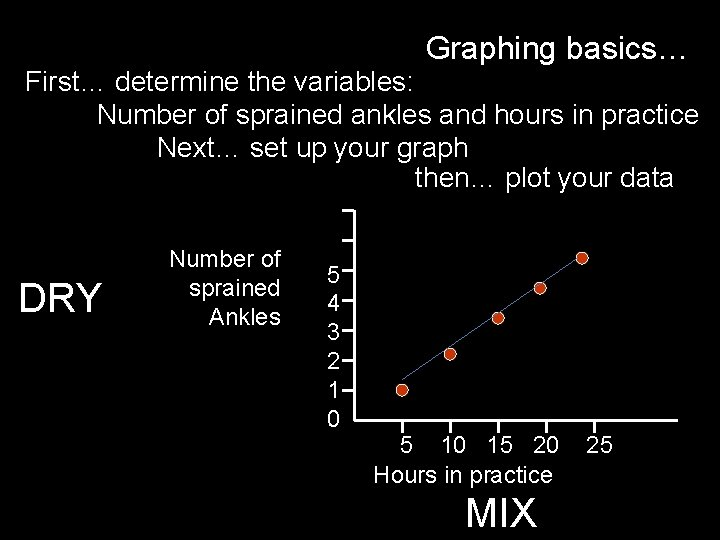Graphing basics… First… determine the variables: Number of sprained ankles and hours in practice Next… set up your graph then… plot your data DRY Number of sprained Ankles 5 4 3 2 1 0 5 10 15 20 Hours in practice MIX 25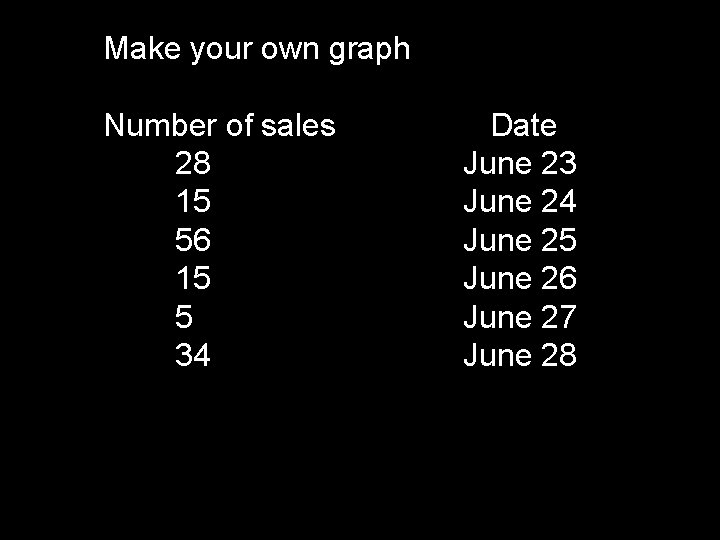Make your own graph Number of sales 28 15 56 15 5 34 Date June 23 June 24 June 25 June 26 June 27 June 28